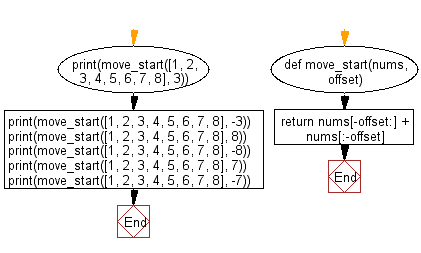﻿ Python: Moves the specified amount of elements to the start of the list - w3resource# Python: Moves the specified amount of elements to the start of the list

## Python List: Exercise - 263 with Solution

Write a Python program to move the specified number of elements to the start of the given list.

Use slice notation to get the two slices of the list and combine them before returning.

Sample Solution:

Python Code:

``````def move_start(nums, offset):
return nums[-offset:] + nums[:-offset]
print(move_start([1, 2, 3, 4, 5, 6, 7, 8], 3))
print(move_start([1, 2, 3, 4, 5, 6, 7, 8], -3))
print(move_start([1, 2, 3, 4, 5, 6, 7, 8], 8))
print(move_start([1, 2, 3, 4, 5, 6, 7, 8], -8))
print(move_start([1, 2, 3, 4, 5, 6, 7, 8], 7))
print(move_start([1, 2, 3, 4, 5, 6, 7, 8], -7))
```
```

Sample Output:

```[6, 7, 8, 1, 2, 3, 4, 5]
[4, 5, 6, 7, 8, 1, 2, 3]
[1, 2, 3, 4, 5, 6, 7, 8]
[1, 2, 3, 4, 5, 6, 7, 8]
[2, 3, 4, 5, 6, 7, 8, 1]
[8, 1, 2, 3, 4, 5, 6, 7]
```

Flowchart:## Visualize Python code execution:

The following tool visualize what the computer is doing step-by-step as it executes the said program:

Python Code Editor:

Have another way to solve this solution? Contribute your code (and comments) through Disqus.

What is the difficulty level of this exercise?

Test your Python skills with w3resource's quiz

﻿

## Python: Tips of the Day

Floor Division:

When we speak of division we normally mean (/) float division operator, this will give a precise result in float format with decimals.

For a rounded integer result there is (//) floor division operator in Python. Floor division will only give integer results that are round numbers.

```print(1000 // 300)
print(1000 / 300)```

Output:

```3
3.3333333333333335```